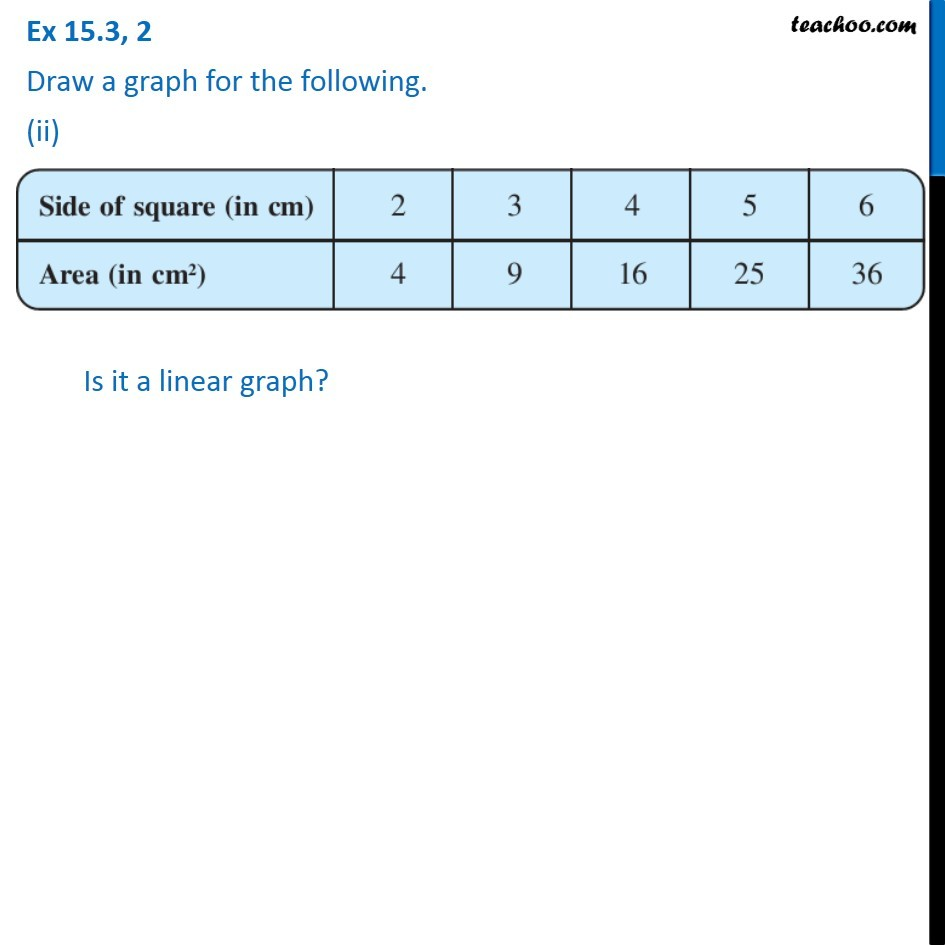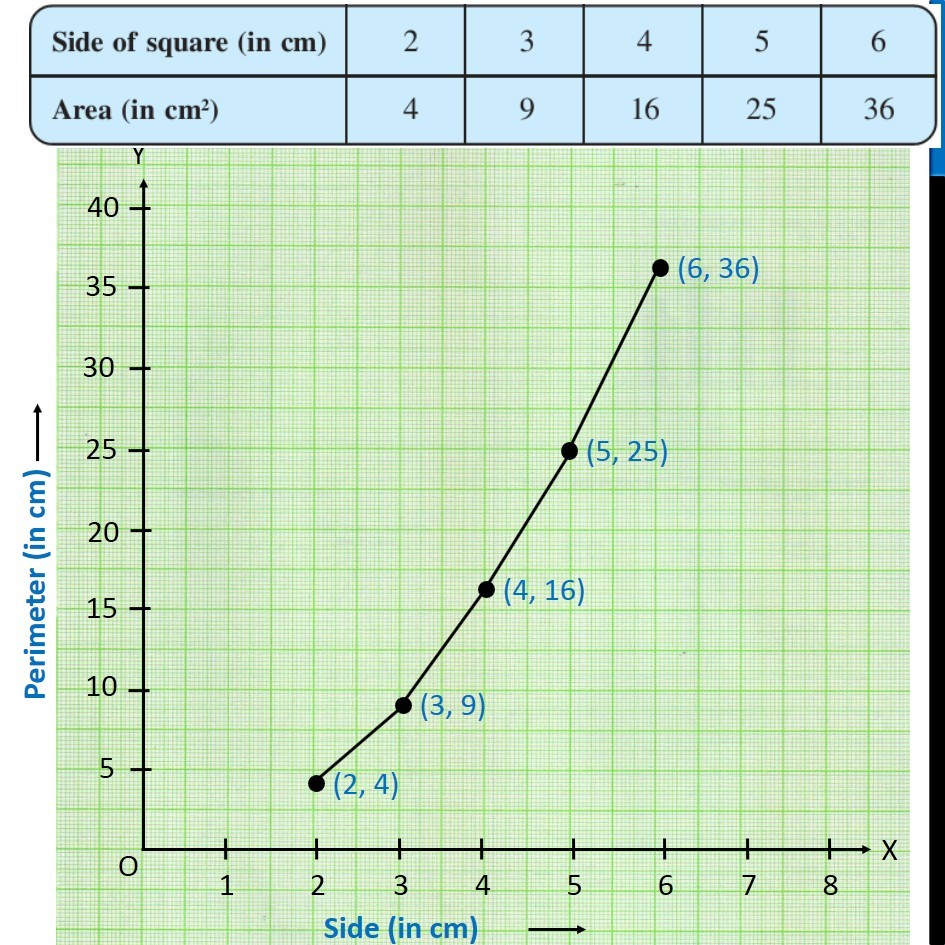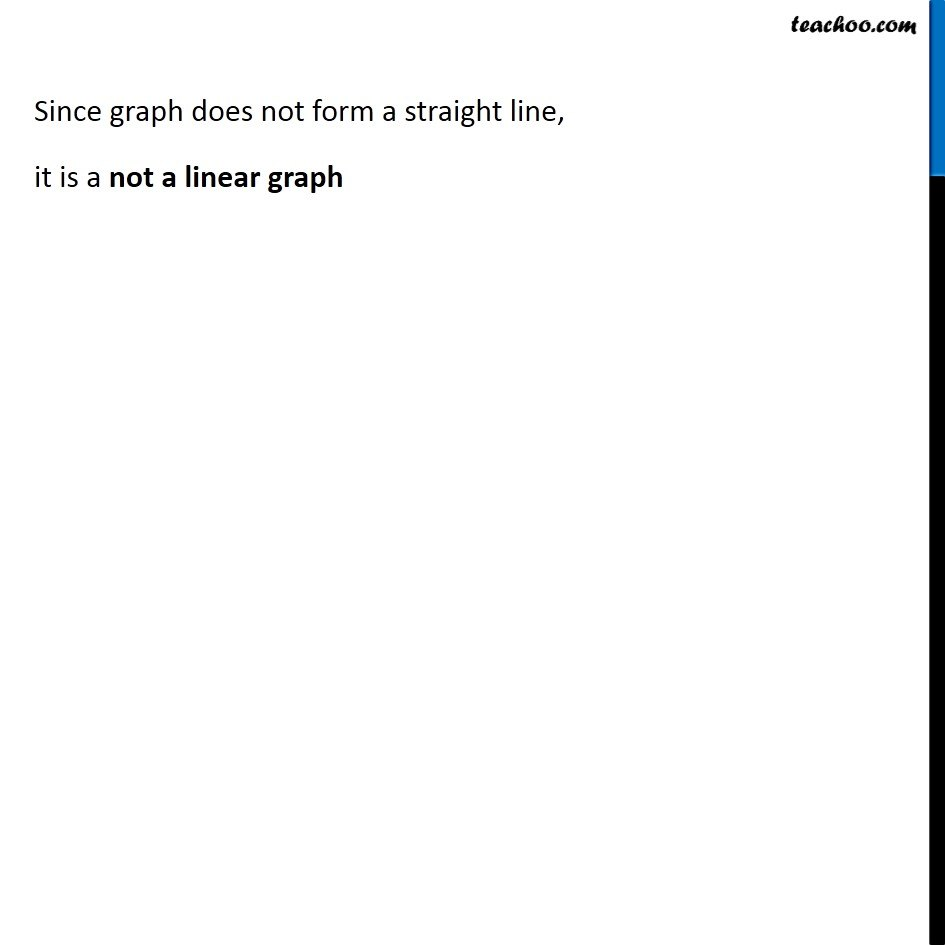1. Chapter 15 Class 8 Introduction to Graphs
2. Concept wise
3. Making Linear Graphs

Transcript

Ex 15.3, 2 Draw a graph for the following. (ii) Side of square (in cm) | 2 | 3 | 4 | 5 | 6 Area (in cm2) | 4 | 9 | 16 | 25 | 36 Is it a linear graph? Plotting Area of square in y-axis, from 0 to 40 (with a gap of 5) and Side of square in x-axis, from 0 to 8 (with a gap of 1) Since graph does not form a straight line, it is a not a linear graph

Making Linear Graphs